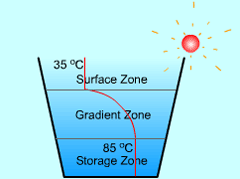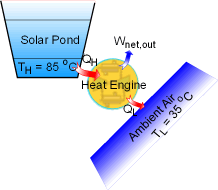Ch 5. Second Law of Thermodynamics Multimedia Engineering Thermodynamics Heat Engine The SecondLaw CarnotCycle Carnot HeatEngine CarnotRefrigerator
 Chapter 1. Basics 2. Pure Substances 3. First Law 4. Energy Analysis 5. Second Law 6. Entropy 7. Exergy Analysis 8. Gas Power Cyc 9. Brayton Cycle 10. Rankine Cycle Appendix Basic Math Units Thermo Tables Search eBooks Dynamics Fluids Math Mechanics Statics Thermodynamics Author(s): Meirong Huang Kurt Gramoll ©Kurt GramollTHERMODYNAMICS - CASE STUDY IntroductionHow a Solar Pond Traps Solar Energy The bottom zone of a pond can be used as a heat source since that zone has a concentrated salt solution with a higher temperature from absorbing solar radiation. This type of pond is called a solar pond. Solar ponds have been used to produce hot water and have good efficiency. A company wants to use this concept to generate power. Hence, a model pond is built to test its performance. What is known: The size of the pond is 10m x 10m x 3m. The ambient air temperature is 35 oC. The temperature at the bottom of the pond is 85 oC. The salt water in the bottom layer absorbs solar radiation at the rate of 0.3 kW/m2. Question Determine the maximum thermal efficiency that this power plant can have. Determine the maximum power generated by each m2 of the bottom of the pond. ApproachHeat Engine Works between the Solar Pond and the Ambient Air The power plant is a heat engine since it generates power at the expense of heat input. Carnot heat engine has the highest thermal efficiency. ηth,rev = 1- TL/TH The thermal efficiency of a heat engine, regardless reversible or irreversible, is given by ηth = 1- QL/QH